## Box Plot Blunders

Amanda Birmingham (abirmingham at ucsd.edu)

It is a truth universally acknowledged that a scientist in possession of a new dataset must be in want of visualization. Unfortunately, while its certainly better to look at our data than not to, choosing the wrong visual summary can impede good analyses by obscuring relevant features while encouraging unwarranted assumptions. In fact, even the friendly and familiar box plot can mislead the unwary!

### Even the friendly and familiar box plot can mislead the unwary, but better options are a simple R command away!

While the limitations of box plots have long been known among statisticians and data scientists, this visualization remains widely used by practicing researchers. Fortunately, box plots’ failings are easy to understand, and better options are a simple R command away.

### Box Plot Basics

Box plots, also sometimes known as box-and-whisker plots, summarize a dataset using a box delimited by the data’s first and third quartiles, broken by a band at the median, and flanked by lines (“whiskers”) representing more outlying data. While the exact meaning of the whiskers can vary, the standard R `boxplot` command and the ggplot2 `geom_boxplot` command both make the whisker length equal to 1.5 * the interquartile range; any data more outlying than that are displayed as individual points. Here’s a basic box plot of 50 random normally-distributed points using ggplot2:

``````library(ggplot2)

numPoints = 1000
testData = data.frame(group = factor(rep(c("A"), each=numPoints)),
measurement = rnorm(numPoints, 50, 15))
ggplot(testData, aes(x=group, y=measurement)) + geom_boxplot()
``````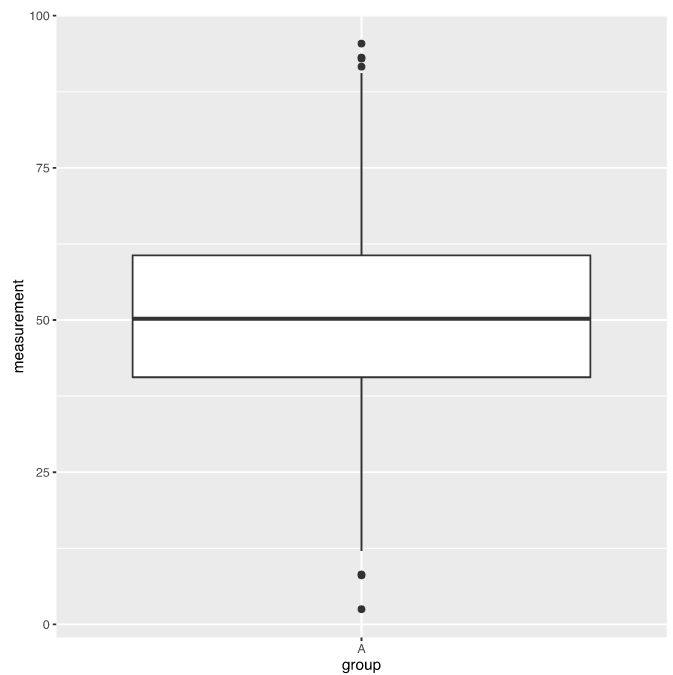Now, using only box plots, compare the data generated above with a new mystery dataset: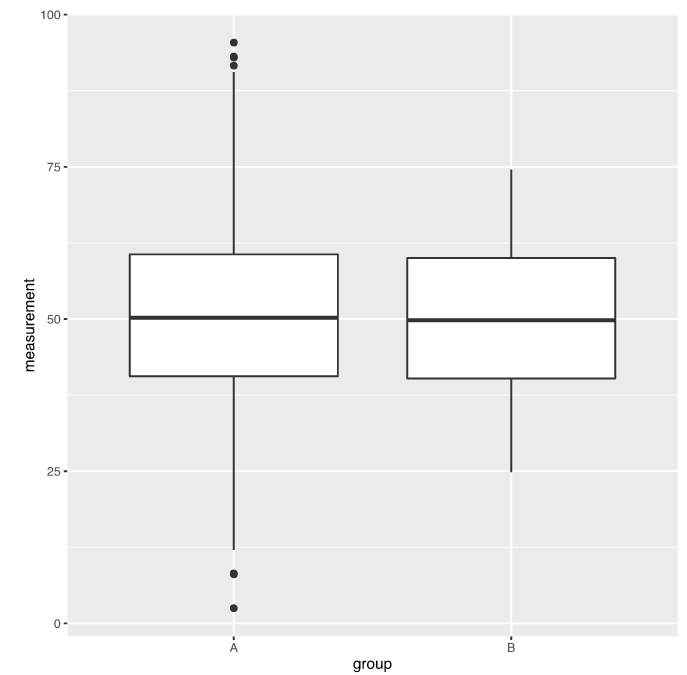What conclusions would you draw? Although the second one has less variation, they look pretty similar …. Might you, for instance, conclude that the second dataset is “normal enough” to compare to the first with a t-test? If so, you’ve fallen prey to a box plot blunder!

### Quartile Statistics Aren’t Enough

Here’s how I generated the group B data in above plot:

``````mysteryData = data.frame(group = factor(rep(c("B"), each=numPoints)),
measurement = c(rnorm(numPoints, 40, 5),
rnorm(numPoints, 60, 5)))
combinedData = rbind(testData, mysteryData)
ggplot(combinedData, aes(x=group, y=measurement)) + geom_boxplot()
``````

For clarity, try visualizing group B a different way:

``````ggplot(combinedData, aes(x=measurement)) + geom_histogram()
``````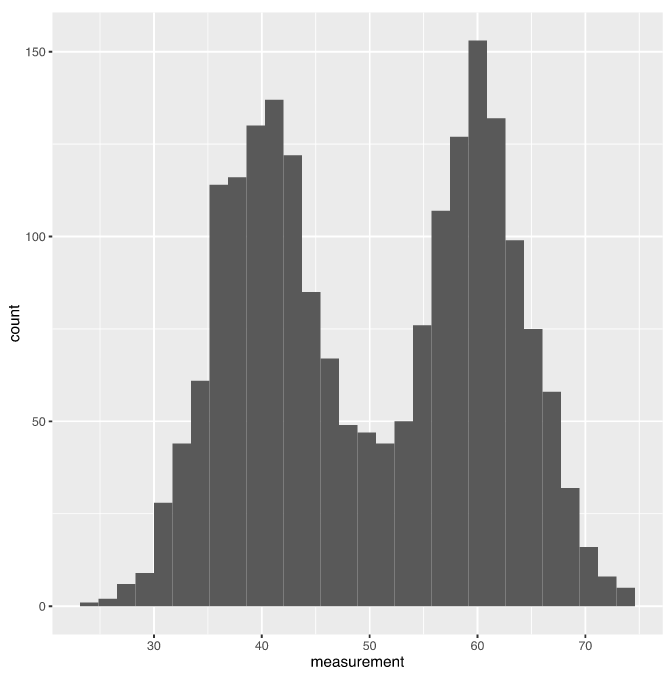Yup, that’s a bimodal distribution! Compare it to group A:

``````ggplot(normalData, aes(x=measurement)) + geom_histogram()
``````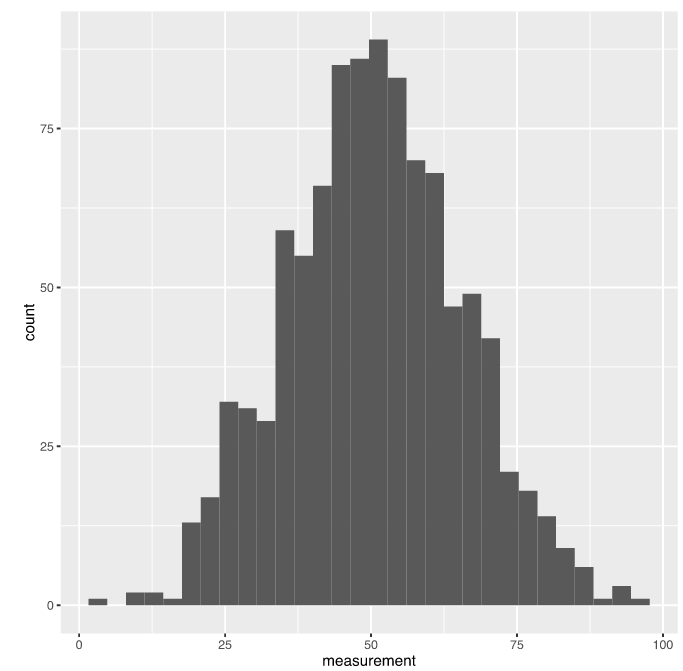The visual similarity between group B’s box plot and that of the normally distributed group A is due to analogous first, second, and third quartile values, but those quartile statistics don’t capture the important distribution information that shows how different these two data sets really are. Histograms show us that distributional info, but aren’t easy to compare when we have more than a couple of groups. What’s a researcher to do?

### The World’s Tiniest Violin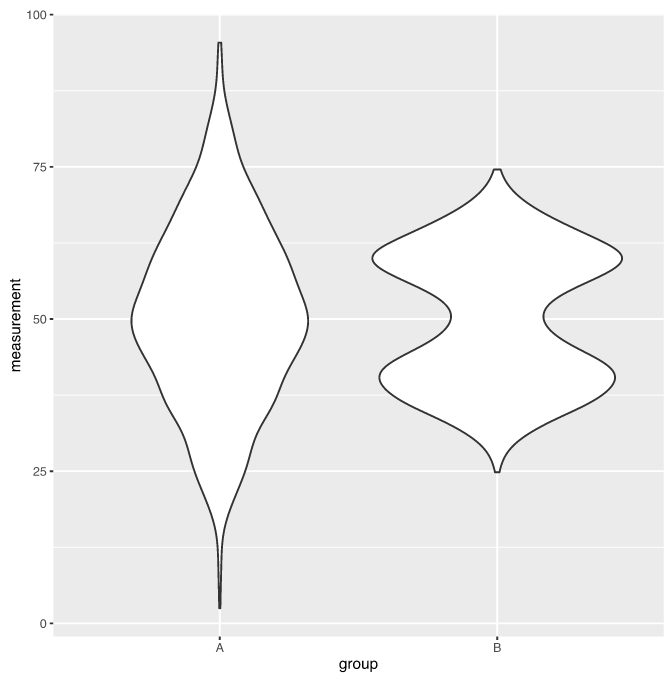This nifty visualization, which fits a smoothed distribution to each dataset, mirrors it around the group’s y axis, and plots all groups along the x axis as is done in box plots, is called a “violin plot”. (The reason for this lyrical name should be readily apparent from, for example, the shape of group B’s plot.) Even the most cursory examination of this graphic makes it clear that these two groups have radically different distributions. Happily, violin plots are built right in to ggplot2 and can be created just as easily as box plots:

``````ggplot(combinedData, aes(x=group, y=measurement)) + geom_violin()
``````

Actually, there’s (at least) one more way in which violin plots can visualize information that is unfortunately hidden by box plots. Scroll back up and look at the y axis scale on the histograms we plotted earlier–what do you see? That’s right: not only do these data sets have different distributions, but they also have very different sizes! Specifically, group B is twice as large as group A. By default, ggplot2 violin plots are scaled to have the same area in each group, but a very small tweak to the command above will instead scale the area to reflect the number of observations in each group:

``````ggplot(combinedData, aes(x=group, y=measurement)) +
geom_violin(scale="count")
``````Now we can easily see both distribution and size information about these datasets. One more little tweak adds the same first-through-third quartile information seen in box plots:

``````ggplot(combinedData, aes(x=group, y=measurement)) +
geom_violin(scale="count", draw_quantiles = c(0.25, 0.5, 0.75))
``````Voila (or perhaps “viola”? Sorry, couldn’t resist the pun!)–goodbye, box plot blunders!

### Beans and Beyond

Of course, violin plots aren’t the only box plot alternatives. Violin plots still disguise the actual data points in favor of smoothed distributions and quartile statistics, unlike another visualization known as the “beanplot”:Beanplots display the smoothed distribution of the data, a single bold line at the average (not median) of the dataset, and also a small line for every data point. (This is called a “beanplot” because its inventor sees the distribution lines as looking like an enclosing bean pod and the individual observation lines as looking like the beans inside.) In larger datasets like the 3000-point test set we’ve been using, these small lines can blend together as shown here, so let’s look instead at some data that is better suited to a beanplot:

``````smallNumPoints = 100
smNormalData = data.frame(group = factor(rep(c("A"), each=smallNumPoints)),
measurement = c(rnorm(smallNumPoints-10, 50, 15),
rep(51, 10)))
smBimodalData = data.frame(group = factor(rep(c("B"), each=smallNumPoints)),
measurement = c(rnorm(smallNumPoints, 40, 5),
rnorm(smallNumPoints, 60, 5)))
combinedSmallData = rbind(smNormalData, smBimodalData)

library(beanplot)
beanplot(measurement ~ group, data=combinedSmallData)
``````This dataset is a tenth the size, allowing us to see individual data points more clearly. I’ve also doped 10 repeated measurements into group A, each with a value of 51; since the beanplot scales each observation line by the number of observations with that value, we can easily see the spike of identical, repeated observations that would be invisible in either a box plot or a violin plot.

Unfortunately, currently beanplots aren’t built into ggplot2 (although they can be pieced together using that library’s extensive options, as shown here) and the stand-alone R library that implements them requires learning different command conventions and so forth. It also doesn’t trim the distributions to end at the most outlying data points, as ggplot2’s violin plot command does by default, and it won’t scale the distributions to the size of the dataset as demonstrated above for violin plots. Still, it offers one extra goodie: asymmetrical plotting.The distribution-mirroring in violin plots is just a visual convention and in my opinion doesn’t really make the data more interpretable. If your data only has two groups (like our test data), the beanplot library allows you to drop the mirroring and instead plotting those groups on two sides of the same axis, as shown above, for easier comparison:

``````beanplot(measurement ~ group, data=combinedSmallData, side = "both",
col = list("black", c("grey", "white")))
``````

Note that the asymmetry comes from the simple `side = "both"` setting; it actually takes more work to set up the colors than the mixed-group display.

### Better Living Through Visualization

Hopefully this little tour has convinced you that box plots are not always trustworthy reporters of a dataset’s salient features–but, frankly, neither are violin plots or beanplots in all circumstances. Fortunately, existing R packages make creating multiple different visualizations from the same data (as done here) a cinch. Take an extra minute or two to look at your data using more than one of them in order to get the full story!

« Return